GeeksforGeeks App
Open AppBrowser
Continue

# How To Make Half Violinplot with ggplot2 in R?

Half Violin plots are basically used to visualize the distribution and the overall summary of the data at the same time. They are also known as Raincloud plots. Combination of half violin plots with jittered points on top, boxplots and can be further enhanced by adding central measures of tendency, quartile ranges etc. Using this plot we can acquire insights about the density, key summary statistics and overall range of the data.

In this article let’s check out how to plot a Half Violin Plot using ggplot2 Package in the R programming language.

## Install and Load the required packages:

Let’s install and load ggplot2 and ggforce packages.

## R

 `# Install and Load the packages`` ` `install.packages``(``"ggplot2"``)``install.packages``(``"ggforce"``)`` ` `library``(ggplot2)``library``(ggforce)`

Let’s load an in-built dataset called diamonds.

## R

 `# Load the diamonds dataset`` ` `df <- diamonds``head``(df)`

Output: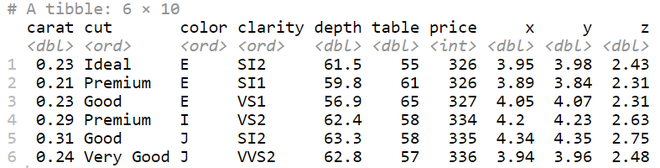## Plotting a Half Violin Plot using ggplot2

### Example 1: Simple Half Violin plot

Let’s plot a Half Violin plot for the cut vs x of diamonds dataset.

## R

 `# simple half violin plot``ggplot``(df, ``aes``(cut , x, fill = cut)) +``geom_flat_violin``() +``theme``(legend.position = ``"none"``)`

Output: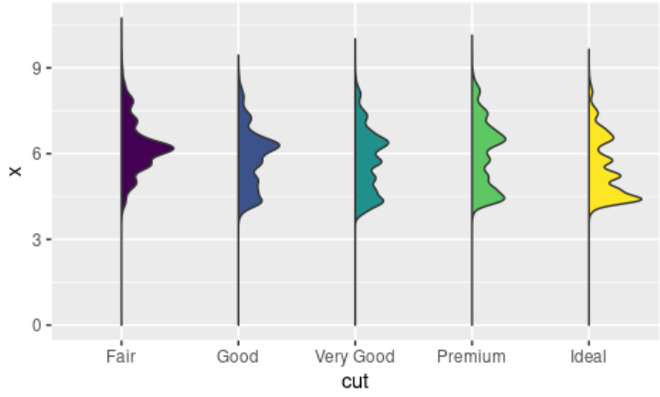### Example 2: Horizontal Half Violin Plot

Let’s check out to align the Half violin plot horizontally using coord_flip() function.

## R

 `# Horizontal half violin plot``ggplot``(df, ``aes``(cut, x, fill = cut)) +``geom_flat_violin``() +``coord_flip``() +``theme``(legend.position = ``"none"``)`

Output: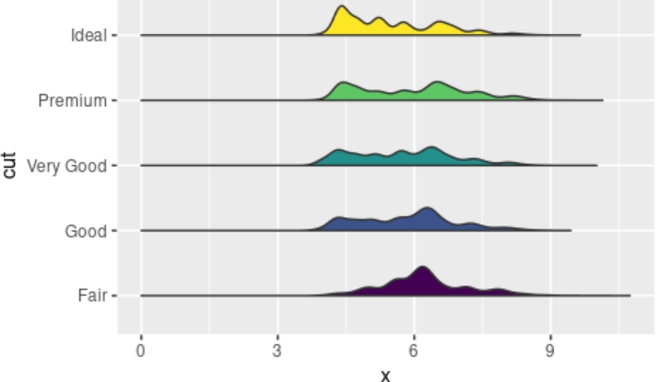### Example 3: Horizontal Half Violin Plot with color filled by cut

Let’s check out to plot a Half violin plot horizontally and fill color by column cut.

## R

 `# Half violin plot with color``ggplot``(df, ``aes``(cut,x, color=cut)) +``geom_flat_violin``() + ``coord_flip``()+``theme``(legend.position = ``"none"``)`

Output: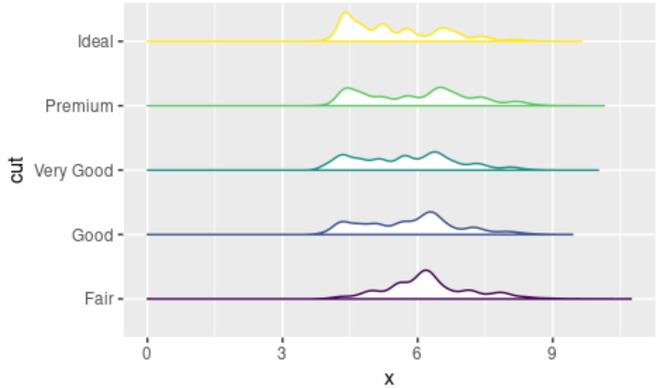### Example 4: Horizontal Half Violin Plot with jittered data points by the side

Let’s check out to plot a Half violin plot along with jittered points.

## R

 `# half violin plot with jittered points``ggplot``(df, ``aes``(cut, x, fill = cut)) +``geom_flat_violin``(position = ``position_nudge``(x = .2, y = 0)) +``geom_jitter``(alpha = 0.01, width = 0.15) +``theme``(legend.position = ``"none"``)`

Output: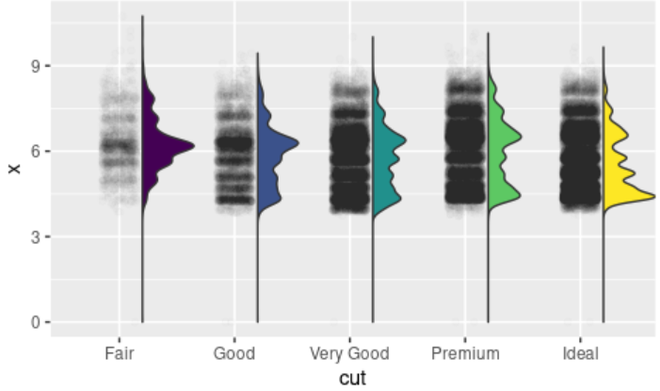My Personal Notes arrow_drop_up